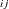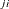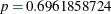# The MODEL Procedure

### Example 19.2 A Consumer Demand Model

This example shows the estimation of a system of nonlinear consumer demand equations based on the translog functional form by using seemingly unrelated regression (SUR). Expenditure shares and corresponding normalized prices are given for three goods.

Since the shares add up to one, the system is singular; therefore, one equation is omitted from the estimation process. The choice of which equation to omit is arbitrary. The nonlinear system is first estimated in unrestricted form by the following statements:

```title1 'Consumer Demand--Translog Functional Form';
title2 'Asymmetric Model';

proc model data=tlog1;
endogenous share1 share2;
parms a1 a2 b11 b12 b13 b21 b22 b23 b31 b32 b33;

bm1 = b11 + b21 + b31;
bm2 = b12 + b22 + b32;
bm3 = b13 + b23 + b33;
lp1 = log(p1);
lp2 = log(p2);
lp3 = log(p3);
share1 = ( a1 + b11 * lp1 + b12 * lp2 + b13 * lp3 ) /
( -1 + bm1 * lp1 + bm2 * lp2 + bm3 * lp3 );
share2 = ( a2 + b21 * lp1 + b22 * lp2 + b23 * lp3 ) /
( -1 + bm1 * lp1 + bm2 * lp2 + bm3 * lp3 );

fit share1 share2
start=( a1 -.14 a2 -.45 b11 .03 b12 .47 b22 .98 b31 .20
b32 1.11 b33 .71 ) / outsused=smatrix sur;
run;
```

A portion of the printed output produced by this example is shown in Output 19.2.1 through Output 19.2.3.

Output 19.2.1: Translog Demand Model Summary

 Consumer Demand--Translog Functional Form Asymmetric Model

The MODEL Procedure

Model Summary
Model Variables 2
Endogenous 2
Parameters 11
Equations 2
Number of Statements 8

Model Variables share1 share2 a1(-0.14) a2(-0.45) b11(0.03) b12(0.47) b13 b21 b22(0.98) b23 b31(0.2) b32(1.11) b33(0.71) share1 share2

The 2 Equations to Estimate
share1 = F(a1, b11, b12, b13, b21, b22, b23, b31, b32, b33)
share2 = F(a2, b11, b12, b13, b21, b22, b23, b31, b32, b33)

Output 19.2.2: Estimation Summary for the Unrestricted Model

 NOTE: At SUR Iteration 2 CONVERGE=0.001 Criteria Met.

 Consumer Demand--Translog Functional Form Asymmetric Model

The MODEL Procedure
SUR Estimation Summary

Data Set Options
DATA= TLOG1
OUTSUSED= SMATRIX

Minimization Summary
Parameters Estimated 11
Method Gauss
Iterations 2

Final Convergence Criteria
R 0.00016
PPC(b11) 0.00116
RPC(b11) 0.012106
Object 2.921E-6
Trace(S) 0.000078
Objective Value 1.749312

Observations
Processed
Solved 44

Output 19.2.3: Estimation Results for the Unrestricted Model

 Consumer Demand--Translog Functional Form Asymmetric Model

The MODEL Procedure

Nonlinear SUR Summary of Residual Errors
Equation DF Model DF Error SSE MSE Root MSE R-Square Adj R-Sq
share1 5.5 38.5 0.00166 0.000043 0.00656 0.8067 0.7841
share2 5.5 38.5 0.00135 0.000035 0.00592 0.9445 0.9380

Nonlinear SUR Parameter Estimates
Parameter Estimate Approx Std Err t Value Approx
Pr > |t|
a1 -0.14881 0.00225 -66.08 <.0001
a2 -0.45776 0.00297 -154.29 <.0001
b11 0.048382 0.0498 0.97 0.3379
b12 0.43655 0.0502 8.70 <.0001
b13 0.248588 0.0516 4.82 <.0001
b21 0.586326 0.2089 2.81 0.0079
b22 0.759776 0.2565 2.96 0.0052
b23 1.303821 0.2328 5.60 <.0001
b31 0.297808 0.1504 1.98 0.0550
b32 0.961551 0.1633 5.89 <.0001
b33 0.8291 0.1556 5.33 <.0001

Number of Observations Statistics for System
Used 44 Objective 1.7493
Missing 0 Objective*N 76.9697

The model is then estimated under the restriction of symmetry (b= b), as shown in the following statements:

```title2 'Symmetric Model';
proc model data=tlog1;
var share1 share2 p1 p2 p3;
parms a1 a2 b11 b12 b22 b31 b32 b33;
bm1 = b11 + b12 + b31;
bm2 = b12 + b22 + b32;
bm3 = b31 + b32 + b33;
lp1 = log(p1);
lp2 = log(p2);
lp3 = log(p3);
share1 = ( a1 + b11 * lp1 + b12 * lp2 + b31 * lp3 ) /
( -1 + bm1 * lp1 + bm2 * lp2 + bm3 * lp3 );
share2 = ( a2 + b12 * lp1 + b22 * lp2 + b32 * lp3 ) /
( -1 + bm1 * lp1 + bm2 * lp2 + bm3 * lp3 );
fit share1 share2
start=( a1 -.14 a2 -.45 b11 .03 b12 .47 b22 .98 b31 .20
b32 1.11 b33 .71 ) / sdata=smatrix sur;
run;
```

A portion of the printed output produced for the symmetry restricted model is shown in Output 19.2.4 and Output 19.2.5.

Output 19.2.4: Model Summary from the Restricted Model

 Consumer Demand--Translog Functional Form Symmetric Model

The MODEL Procedure

The 2 Equations to Estimate
share1 = F(a1, b11, b12, b22, b31, b32, b33)
share2 = F(a2, b11, b12, b22, b31, b32, b33)

Output 19.2.5: Estimation Results for the Restricted Model

 Consumer Demand--Translog Functional Form Symmetric Model

The MODEL Procedure

Nonlinear SUR Summary of Residual Errors
Equation DF Model DF Error SSE MSE Root MSE R-Square Adj R-Sq
share1 4 40 0.00166 0.000041 0.00644 0.8066 0.7920
share2 4 40 0.00139 0.000035 0.00590 0.9428 0.9385

Nonlinear SUR Parameter Estimates
Parameter Estimate Approx Std Err t Value Approx
Pr > |t|
a1 -0.14684 0.00135 -108.99 <.0001
a2 -0.4597 0.00167 -275.34 <.0001
b11 0.02886 0.00741 3.89 0.0004
b12 0.467827 0.0115 40.57 <.0001
b22 0.970079 0.0177 54.87 <.0001
b31 0.208143 0.00614 33.88 <.0001
b32 1.102415 0.0127 86.51 <.0001
b33 0.694245 0.0168 41.38 <.0001

Number of Observations Statistics for System
Used 44 Objective 1.7820
Missing 0 Objective*N 78.4097

Hypothesis testing requires that the S matrix from the unrestricted model be imposed on the restricted model, as explained in the section Tests on Parameters. The S matrix saved in the data set SMATRIX is requested by the SDATA= option.

A chi-square test is used to see if the hypothesis of symmetry is accepted or rejected. (Oc–Ou ) has a chi-square distribution asymptotically, where Oc is the constrained OBJECTIVE*N and Ou is the unconstrained OBJECTIVE*N. The degrees of freedom is equal to the difference in the number of free parameters in the two models.

In this example, Ou is 76.9697 and Oc is 78.4097, resulting in a difference of 1.44 with 3 degrees of freedom. You can obtain the probability value by using the following statements:

```data _null_;
/* probchi( reduced-full, n-restrictions )*/
p = 1-probchi( 1.44, 3 );
put p=;
run;
```

The output from this DATA step run is. With this p-value you cannot reject the hypothesis of symmetry. This test is asymptotically valid.